# bert:深入理解## BERT 预训练模型及文本分类

#### 知识点

• 语言模型和词向量

• BERT 结构详解

• BERT 文本分类

BERT 全称为 Bidirectional Encoder Representations from Transformer，是谷歌在 2018 年 10 月发布的语言表示模型。BERT 通过维基百科和书籍语料组成的庞大语料进行了预训练，使用时只要根据下游任务进行输出层的修改和模型微调训练，就可以得到很好的效果。BERT 发布之初，就在 GLUE、MultiNLI、SQuAD 等评价基准和数据集上取得了超越当时最好成绩的结果。但在深入了解 BERT 结构之前，先需要了解一下什么是语言模型，以及在 BERT 诞生之前人们是如何进行文本向量化的。

### 语言模型和词向量

#### 神经网络语言模型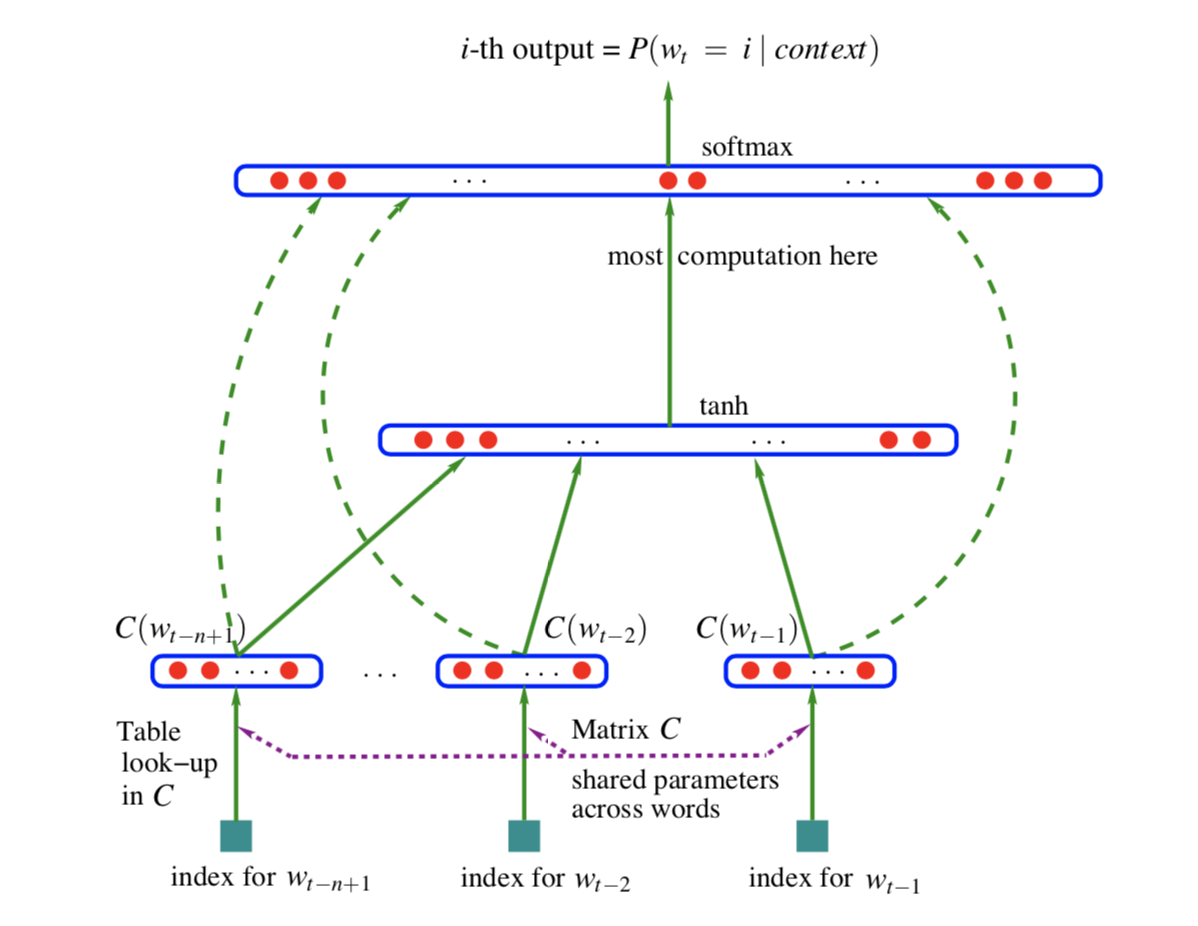### BERT 结构详解

BERT 的整体结构如下图所示，其是以 Transformer 为基础构建的，使用 WordPiece 的方法进行数据预处理，最后通过 MLM 任务和下个句子预测任务进行预训练的语言表示模型。下面我们从 BERT 的结构：Transformer 出发，来一步步详细解析一下 BERT。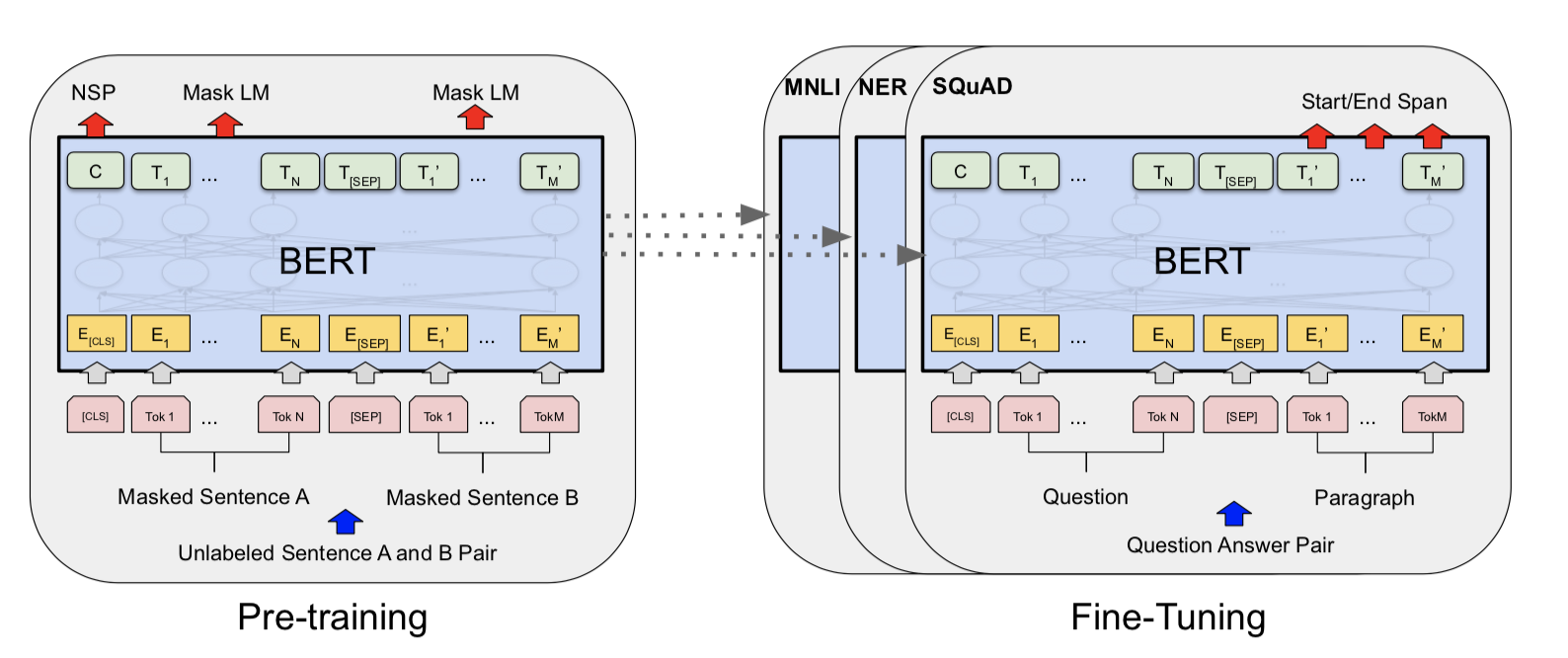#### Transformer

Transformer 的具体结构如下图：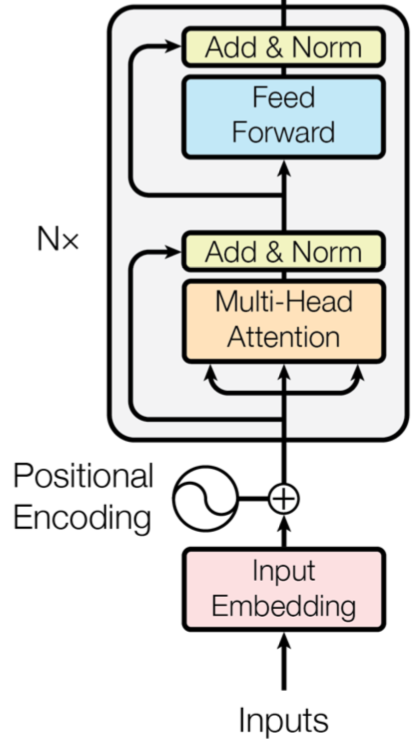Positional Encoding，从字面意思来讲是位置编码，就是用来表示输入句子向量中每个字词所对应的位置。由于 Tranformer 的结构不同，无法像 RNN 一样获取句子的时序信息，所以需要使用 Positional Encoding 表示字词在句子中的先后顺序。一种常见的计算方式是使用正弦函数和余弦函数来构造每个位置的值，后来的研究发现通过可训练的参数来实现的也能够达到同样的效果，BERT 模型中就是通过可训练参数的方法来实现的。

!(C:\Users\jie\Documents\notebook\PyTorch\BERT 预训练模型及文本分类\1.png)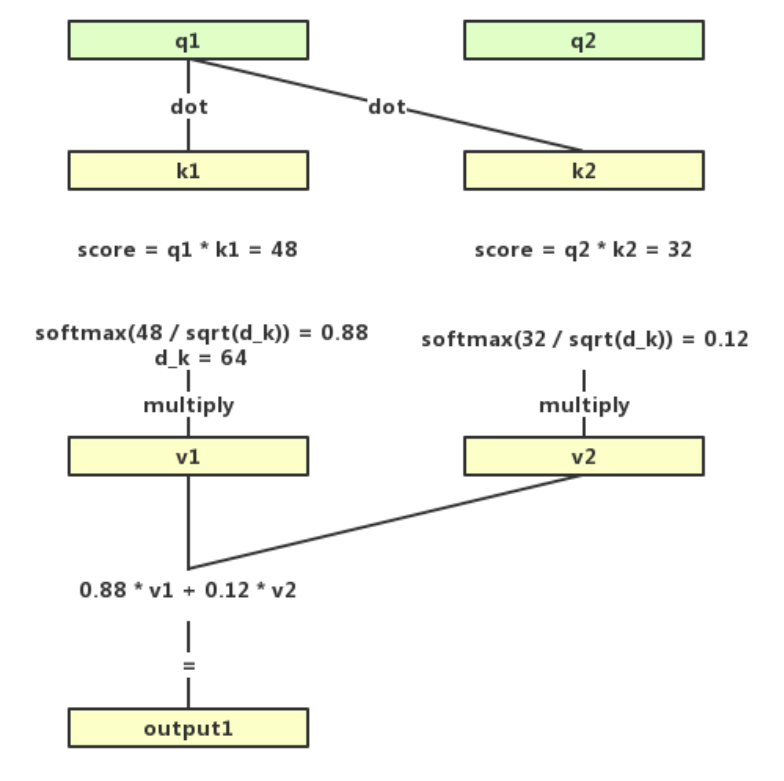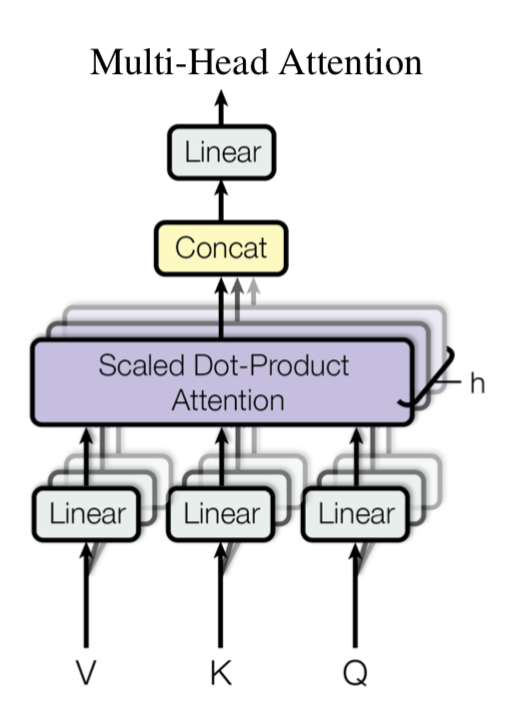```# 取自 hugging face 团队实现的基于 pytorch 的 BERT 模型class BERTSelfAttention(nn.Module):
# BERT 的 Self-Attention 类
def __init__(self, config):
# 初始化函数
super(BERTSelfAttention, self).__init__()        if config.hidden_size % config.num_attention_heads != 0:            raise ValueError(                "The hidden size (%d) is not a multiple of the number of attention "

self.value = nn.Linear(config.hidden_size, self.all_head_size)    def transpose_for_scores(self, x):
x = x.view(*new_x_shape)        return x.permute(0, 2, 1, 3)    def forward(self, hidden_states):
# 前向传播函数
mixed_query_layer = self.query(hidden_states)
mixed_key_layer = self.key(hidden_states)
mixed_value_layer = self.value(hidden_states)

query_layer = self.transpose_for_scores(mixed_query_layer)
key_layer = self.transpose_for_scores(mixed_key_layer)
value_layer = self.transpose_for_scores(mixed_value_layer)        # 将"query"和"key"点乘，得到未经处理注意力值
attention_scores = torch.matmul(query_layer, key_layer.transpose(-1, -2))
attention_scores = attention_scores / math.sqrt(self.attention_head_size)        # 使用 softmax 函数将注意力值标准化成概率值
attention_probs = nn.Softmax(dim=-1)(attention_scores)

context_layer = torch.matmul(attention_probs, value_layer)
context_layer = context_layer.permute(0, 2, 1, 3).contiguous()
context_layer = context_layer.view(*new_context_layer_shape)        return context_layer```

### BERT 预训练模型

#### 遮蔽语言模型

BERT 的论文中提到，增加掩膜的具体方式为：先对语句进行 WordPiece 分割，分割后选择句中 15% 的字符，例如选择到了第 ii 字符，接下来：

1. 以 80%80% 的概率使用 `[Mask]` 替换。

2. 以 10%10% 的概率使用一个随机的字符替换。

3. 以 10%10% 的概率不进行操作。

`!pip install pytorch-transformers==1.0  # 安装 PyTorch-Transformers`

PyTorch-Transformers 是一个以 PyTorch 深度学习框架为基础构建的自然语言处理预训练模型库，早前称之为 `pytorch-pretrained-bert`，如果已正式成为独立项目。

```import torchfrom pytorch_transformers import BertTokenizer

model_name = 'bert-base-chinese'  # 指定需下载的预训练模型参数# BERT 在预训练中引入了 [CLS] 和 [SEP] 标记句子的开头和结尾samples = ['[CLS] 中国的首都是哪里？ [SEP] 北京是 [MASK] 国的首都。 [SEP]']  # 准备输入模型的语句tokenizer = BertTokenizer.from_pretrained(model_name)
tokenized_text = [tokenizer.tokenize(i) for i in samples]
input_ids = [tokenizer.convert_tokens_to_ids(i) for i in tokenized_text]
input_ids = torch.LongTensor(input_ids)
input_ids```

```from pytorch_transformers import BertForMaskedLM# 读取预训练模型model = BertForMaskedLM.from_pretrained(model_name, cache_dir="./")
model.eval()```

```outputs = model(input_ids)
prediction_scores = outputs
prediction_scores.shape```

```import numpy as np

sample = prediction_scores.detach().numpy()
pred = np.argmax(sample, axis=1)

tokenizer.convert_ids_to_tokens(pred)```

`中`

#### 句子预测任务

```samples = ["[CLS]今天天气怎么样？[SEP]今天天气很好。[SEP]", "[CLS]小明今年几岁了？[SEP]小明爱吃西瓜。[SEP]"]
tokenizer = BertTokenizer.from_pretrained(model_name)
tokenized_text = [tokenizer.tokenize(i) for i in samples]
input_ids = [tokenizer.convert_tokens_to_ids(i) for i in tokenized_text]
input_ids = torch.LongTensor(input_ids)
input_ids```

```segments_ids = [[0, 0, 0, 0, 0, 0, 0, 0, 0, 0, 1, 1, 1, 1, 1, 1, 1, 1],
[0, 0, 0, 0, 0, 0, 0, 0, 0, 0, 1, 1, 1, 1, 1, 1, 1, 1]]

segments_tensors = torch.tensor(segments_ids)
segments_tensors```

```from pytorch_transformers import BertForNextSentencePrediction

model = BertForNextSentencePrediction.from_pretrained(
model_name, cache_dir="./")
model.eval()```

```outputs = model(input_ids)
seq_relationship_scores = outputs
seq_relationship_scores```
```sample = seq_relationship_scores.detach().numpy()
pred = np.argmax(sample, axis=1)
pred```

`0` 表示是上下句关系，`1` 表示不是上下句关系。因此从上面结果可以看到，模型预测第一个句子对是上下句关系，第二个句子对不是，对于这两个样本 BERT 的预测正确。

1. 使用的双向的 Transformer 结构学习到左、右两侧上下文语境。

2. 使用完整的文档语料训练而不是打乱的句子，配合下个句子预测任务，从而学习到了捕捉很长的连续语句中的信息的能力。

### BERT 文本分类实践

1. 从预训练好的 BERT 模型中提取特征向量，即 Feature Extraction 方法。

2. 将下游任务模型添加到 BERT 模型之后，然后使用下游任务的训练集对进行训练，即 Fine-Tuning 方法。

```with open('./negdata.txt', 'r', encoding='utf-8') as f:
neg_data = f.read()with open('./posdata.txt', 'r', encoding='utf-8') as f:

neg_datalist = neg_data.split('\n')
pos_datalist = pos_data.split('\n')
len(neg_datalist), len(pos_datalist)```

```import numpy as np

dataset = np.array(pos_datalist + neg_datalist)
labels = np.array( * len(pos_datalist) +  * len(neg_datalist))len(dataset)  # 共 3000 条数据```

```np.random.seed(10)
mix_index = np.random.choice(3000, 3000)
dataset = dataset[mix_index]
labels = labels[mix_index]len(dataset), len(labels)```

```TRAINSET_SIZE = 2500EVALSET_SIZE = 500train_samples = dataset[:TRAINSET_SIZE]  # 2500 条数据train_labels = labels[:TRAINSET_SIZE]
eval_samples = dataset[TRAINSET_SIZE:TRAINSET_SIZE+EVALSET_SIZE]  # 500 条数据eval_labels = labels[TRAINSET_SIZE:TRAINSET_SIZE+EVALSET_SIZE]len(train_samples), len(eval_samples)```

```def get_dummies(l, size=2):
res = list()
for i in l:
tmp =  * size
tmp[i] = 1
res.append(tmp)
return res```

```from torch.utils.data import DataLoader, TensorDataset

tokenized_text = [tokenizer.tokenize(i) for i in train_samples]
input_ids = [tokenizer.convert_tokens_to_ids(i) for i in tokenized_text]
input_labels = get_dummies(train_labels)  # 使用 get_dummies 函数转换标签for j in range(len(input_ids)):    # 将样本数据填充至长度为 512
i = input_ids[j]
if len(i) != 512:
input_ids[j].extend(*(512 - len(i)))# 构建数据集和数据迭代器，设定 batch_size 大小为 4train_set = TensorDataset(torch.LongTensor(input_ids),

torch.FloatTensor(input_labels))
batch_size=4,
shuffle=True)

```tokenized_text = [tokenizer.tokenize(i) for i in eval_samples]
input_ids = [tokenizer.convert_tokens_to_ids(i) for i in tokenized_text]
input_labels = eval_labels
for j in range(len(input_ids)):
i = input_ids[j]
if len(i) != 512:
input_ids[j].extend(*(512 - len(i)))

eval_set = TensorDataset(torch.LongTensor(input_ids),torch.FloatTensor(input_labels))

```device = torch.device("cuda" if torch.cuda.is_available() else "cpu")
device```

```import torch.nn as nn
import torch.nn.functional as F
from pytorch_transformers import BertModel
class fn_cls(nn.Module):
def __init__(self):
super(fn_cls, self).__init__()
self.model = BertModel.from_pretrained(model_name, cache_dir="./")
self.model.to(device)
self.dropout = nn.Dropout(0.1)
self.l1 = nn.Linear(768, 2)
x = outputs  # 取池化后的结果 batch * 768
x = x.view(-1, 768)
x = self.dropout(x)
x = self.l1(x)        return x```

```from torch import optim

cls = fn_cls()
cls.to(device)
cls.train()

criterion = nn.BCELoss()
sigmoid = nn.Sigmoid()

```def predict(logits):
res = torch.argmax(logits, 1)
return res```

```from torch.autograd import Variable
import time

pre = time.time()

accumulation_steps = 8
epoch = 3
for i in range(epoch):
for batch_idx, (data, target) in enumerate(train_loader):
data, target = Variable(data).to(device), Variable(target.view(-1, 2)).to(device)

for sample in data:
mask.append([1 if i != 0 else 0 for i in sample])

pred = predict(output)

loss = criterion(sigmoid(output).view(-1, 2), target)        # 梯度积累
loss = loss/accumulation_steps
loss.backward()
if((batch_idx+1) % accumulation_steps) == 0:            # 每 8 次更新一下网络中的参数
optimizer.step()
if ((batch_idx+1) % accumulation_steps) == 1:
print('Train Epoch: {} [{}/{} ({:.0f}%)]\tLoss:{:.6f}'.format(
))
if batch_idx == len(train_loader)-1:            # 在每个 Epoch 的最后输出一下结果
print('labels:', target)
print('pred:', pred)

print('训练时间：', time.time()-pre)```

```from tqdm import tqdm_notebook as tqdm

cls.eval()

correct = 0total = 0
for batch_idx, (data, target) in enumerate(tqdm(eval_loader)):
data = data.to(device)
target = target.long().to(device)

for sample in data:
mask.append([1 if i != 0 else 0 for i in sample])

pred = predict(output)

correct += (pred == target).sum().item()
total += len(data)# 准确率应该达到百分之 90 以上print('正确分类的样本数：{}，样本总数：{}，准确率：{:.2f}%'.format(
correct, total, 100.*correct/total))```

`test_samples = ['东西很好，好评！', '东西不好，差评！']`
```cls.eval()
tokenized_text = [tokenizer.tokenize(i) for i in test_samples]
input_ids = [tokenizer.convert_tokens_to_ids(i) for i in tokenized_text]
input_ids = torch.LongTensor(input_ids).cuda()

pred = predict(output)
pred```

### 总结https://github.com/zeus-y/